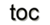Next: 1 Introduction Up: Dissertation Rene Heinzl Previous: List of Figures

# Notation

Due to the wide variety of material in this work drawn from different fields in scientific computing, some conflicts or ambiguitites in the notation cannot be avoided. The following table summarized the used notations: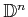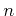-dimensional closed unit ball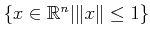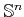-dimensional unit sphere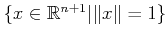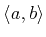scalar product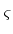of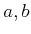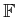field, e.g.,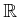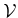vector space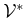dual vector space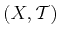topological space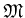manifolddomain, subset of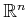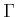boundary of a domain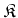cell complex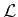linear operator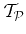tangent space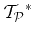cotangent space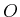(globally) ordered set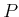partially ordered set (poset)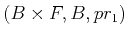trivial fiber bundle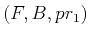fiber bundle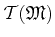tangent bundleconnection matrix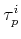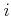-th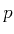-cell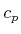-chain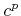-cochain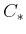chain complex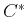cochain complex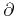boundary operator, partial derivative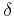coboundary operator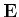electric field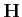magnetic field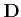electric flux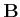magnetic flux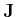current flux density vector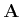magnetic vector potential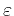dielectric permittivity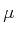magnetic permeability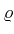electrical charge density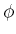basis function for finite elements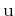generic solution quantityNext: 1 Introduction Up: Dissertation Rene Heinzl Previous: List of Figures

R. Heinzl: Concepts for Scientific Computing